# 6.3 Inverse trigonometric functions  (Page 8/15)

 Page 8 / 15

The line $\text{\hspace{0.17em}}y=\frac{3}{5}x\text{\hspace{0.17em}}$ passes through the origin in the x , y -plane. What is the measure of the angle that the line makes with the positive x -axis?

The line $\text{\hspace{0.17em}}y=\frac{-3}{7}x\text{\hspace{0.17em}}$ passes through the origin in the x , y -plane. What is the measure of the angle that the line makes with the negative x -axis?

What percentage grade should a road have if the angle of elevation of the road is 4 degrees? (The percentage grade is defined as the change in the altitude of the road over a 100-foot horizontal distance. For example a 5% grade means that the road rises 5 feet for every 100 feet of horizontal distance.)

A 20-foot ladder leans up against the side of a building so that the foot of the ladder is 10 feet from the base of the building. If specifications call for the ladder's angle of elevation to be between 35 and 45 degrees, does the placement of this ladder satisfy safety specifications?

No. The angle the ladder makes with the horizontal is 60 degrees.

Suppose a 15-foot ladder leans against the side of a house so that the angle of elevation of the ladder is 42 degrees. How far is the foot of the ladder from the side of the house?

## Graphs of the Sine and Cosine Functions

For the following exercises, graph the functions for two periods and determine the amplitude or stretching factor, period, midline equation, and asymptotes.

$f\left(x\right)=-3\mathrm{cos}\text{\hspace{0.17em}}x+3$

amplitude: 3; period: $\text{\hspace{0.17em}}2\pi ;\text{\hspace{0.17em}}$ midline: $\text{\hspace{0.17em}}y=3;\text{\hspace{0.17em}}$ no asymptotes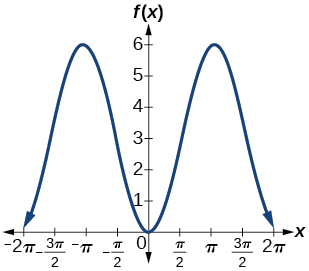$f\left(x\right)=\frac{1}{4}\mathrm{sin}\text{\hspace{0.17em}}x$

$f\left(x\right)=3\mathrm{cos}\left(x+\frac{\pi }{6}\right)$

amplitude: 3; period: $\text{\hspace{0.17em}}2\pi ;\text{\hspace{0.17em}}$ midline: $\text{\hspace{0.17em}}y=0;\text{\hspace{0.17em}}$ no asymptotes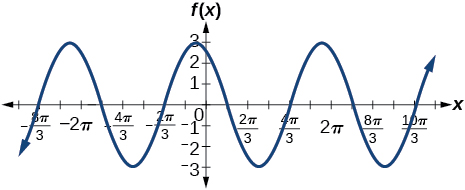$f\left(x\right)=-2\mathrm{sin}\left(x-\frac{2\pi }{3}\right)$

$f\left(x\right)=3\mathrm{sin}\left(x-\frac{\pi }{4}\right)-4$

amplitude: 3; period: $\text{\hspace{0.17em}}2\pi ;\text{\hspace{0.17em}}$ midline: $\text{\hspace{0.17em}}y=-4;\text{\hspace{0.17em}}$ no asymptotes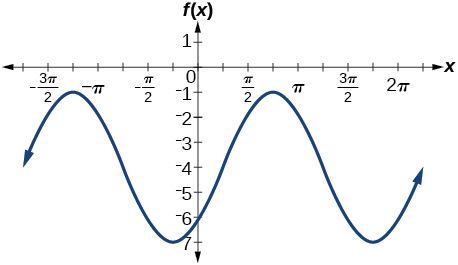$f\left(x\right)=2\left(\mathrm{cos}\left(x-\frac{4\pi }{3}\right)+1\right)$

$f\left(x\right)=6\mathrm{sin}\left(3x-\frac{\pi }{6}\right)-1$

amplitude: 6; period: $\text{\hspace{0.17em}}\frac{2\pi }{3};\text{\hspace{0.17em}}$ midline: $\text{\hspace{0.17em}}y=-1;\text{\hspace{0.17em}}$ no asymptotes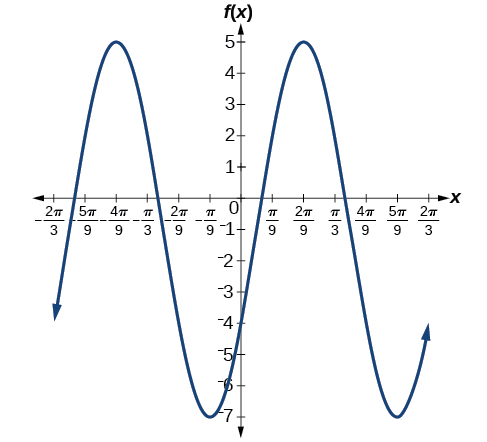$f\left(x\right)=-100\mathrm{sin}\left(50x-20\right)$

## Graphs of the Other Trigonometric Functions

For the following exercises, graph the functions for two periods and determine the amplitude or stretching factor, period, midline equation, and asymptotes.

$f\left(x\right)=\mathrm{tan}\text{\hspace{0.17em}}x-4$

stretching factor: none; period: midline: asymptotes: where is an integer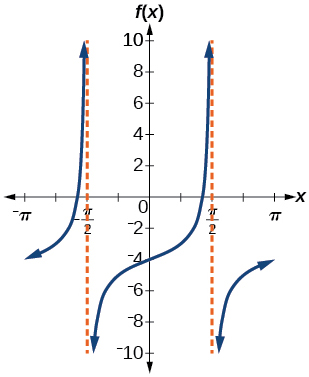$f\left(x\right)=2\mathrm{tan}\left(x-\frac{\pi }{6}\right)$

$f\left(x\right)=-3\mathrm{tan}\left(4x\right)-2$

stretching factor: 3; period: midline: asymptotes: $x=\frac{\pi }{8}+\frac{\pi }{4}k,$ where is an integer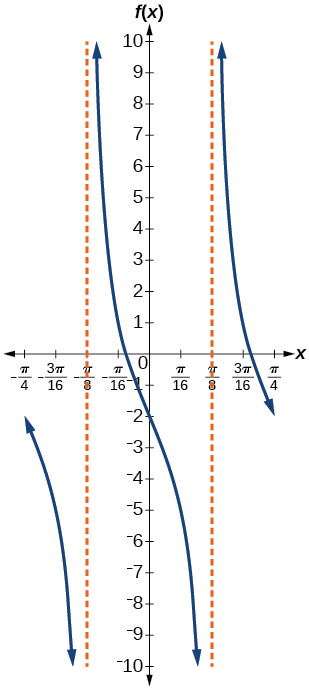$f\left(x\right)=0.2\mathrm{cos}\left(0.1x\right)+0.3$

For the following exercises, graph two full periods. Identify the period, the phase shift, the amplitude, and asymptotes.

$f\left(x\right)=\frac{1}{3}\mathrm{sec}\text{\hspace{0.17em}}x$

amplitude: none; period: $2\pi ;$ no phase shift; asymptotes: where is an odd integer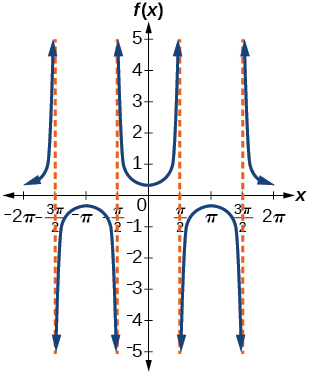$f\left(x\right)=3\mathrm{cot}\text{\hspace{0.17em}}x$

$f\left(x\right)=4\mathrm{csc}\left(5x\right)$

amplitude: none; period: no phase shift; asymptotes: where is an integer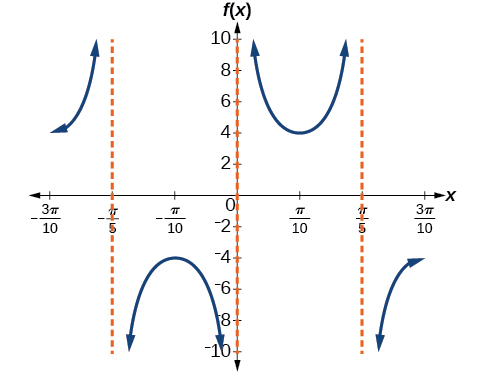$f\left(x\right)=8\mathrm{sec}\left(\frac{1}{4}x\right)$

$f\left(x\right)=\frac{2}{3}\mathrm{csc}\left(\frac{1}{2}x\right)$

amplitude: none; period: no phase shift; asymptotes: where is an integer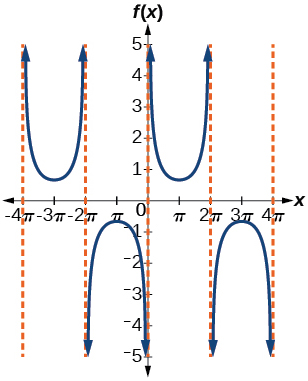$f\left(x\right)=-\mathrm{csc}\left(2x+\pi \right)$

For the following exercises, use this scenario: The population of a city has risen and fallen over a 20-year interval. Its population may be modeled by the following function: $\text{\hspace{0.17em}}y=12,000+8,000\mathrm{sin}\left(0.628x\right),\text{\hspace{0.17em}}$ where the domain is the years since 1980 and the range is the population of the city.

What is the largest and smallest population the city may have?

largest: 20,000; smallest: 4,000

Graph the function on the domain of $\text{\hspace{0.17em}}\left[0,40\right]$ .

difference between calculus and pre calculus?
give me an example of a problem so that I can practice answering
x³+y³+z³=42
Robert
dont forget the cube in each variable ;)
Robert
of she solves that, well ... then she has a lot of computational force under her command ....
Walter
what is a function?
I want to learn about the law of exponent
explain this
what is functions?
A mathematical relation such that every input has only one out.
Spiro
yes..it is a relationo of orders pairs of sets one or more input that leads to a exactly one output.
Mubita
Is a rule that assigns to each element X in a set A exactly one element, called F(x), in a set B.
RichieRich
If the plane intersects the cone (either above or below) horizontally, what figure will be created?
can you not take the square root of a negative number
No because a negative times a negative is a positive. No matter what you do you can never multiply the same number by itself and end with a negative
lurverkitten
Actually you can. you get what's called an Imaginary number denoted by i which is represented on the complex plane. The reply above would be correct if we were still confined to the "real" number line.
Liam
Suppose P= {-3,1,3} Q={-3,-2-1} and R= {-2,2,3}.what is the intersection
can I get some pretty basic questions
In what way does set notation relate to function notation
Ama
is precalculus needed to take caculus
It depends on what you already know. Just test yourself with some precalculus questions. If you find them easy, you're good to go.
Spiro
the solution doesn't seem right for this problem
what is the domain of f(x)=x-4/x^2-2x-15 then
x is different from -5&3
Seid
All real x except 5 and - 3
Spiro
***youtu.be/ESxOXfh2Poc
Loree
how to prroved cos⁴x-sin⁴x= cos²x-sin²x are equal
Don't think that you can.
Elliott
By using some imaginary no.
Tanmay
how do you provided cos⁴x-sin⁴x = cos²x-sin²x are equal
What are the question marks for?
ElliottByByBy Madison ChristianBy OpenStaxBy Stephen VoronBy Sebastian Sieczko...By OpenStaxBy Subramanian DivyaBy OpenStaxBy Alec MoffitBy OpenStaxBy Mucho Mizinduko